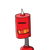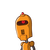# A boy is flying a kite with a string of length 100 m . If the string is tight and the angle of elevation of the kite is 26° 32′ ,

A boy is flying a kite with a string of length 100 m . If the string is tight and the angle of elevation of the kite is 26° 32′ , find the height of the kite correct to one decimal place ( ignore the height of the boy ) .​

### 2 thoughts on “A boy is flying a kite with a string of length 100 m . If the string is tight and the angle of elevation of the kite is 26° 32′ ,”

1.2.• the height of kite is 44.7m

### ToFind:–

• the height of the kite correct to one decimal place.

### Given:–

• Angle of elevation of the kite = 26° 32′

• lenght of string = 100m

### Consider:–

• AB as the height of the kite A and AC as the string,

• → AC = 100
• → Take AB = x

### In right triangle ABC

• => Sin∅ = $$\frac{AB}{AC}$$

$$\:\:\:Substituting\:the\:values,$$

• ⇒sin26° 32′ = $$\frac{x}{100}$$
• ⇒0.4467 = $$\frac{x}{100}$$
• ⇒x = 100 × 0.4467
• ⇒x = 44.67 ≈ 44.7m